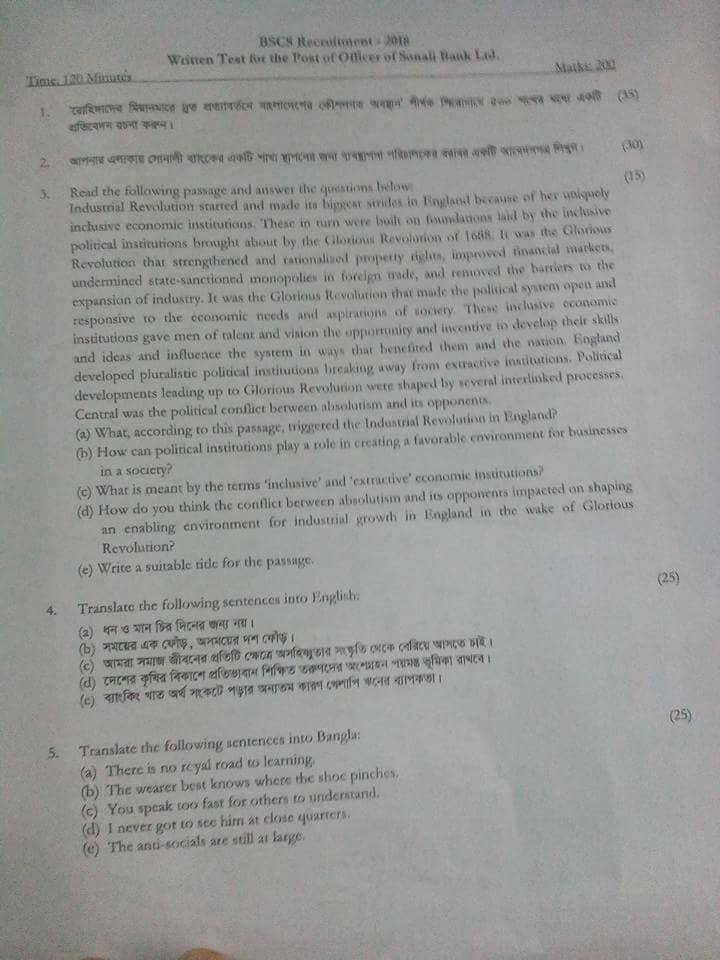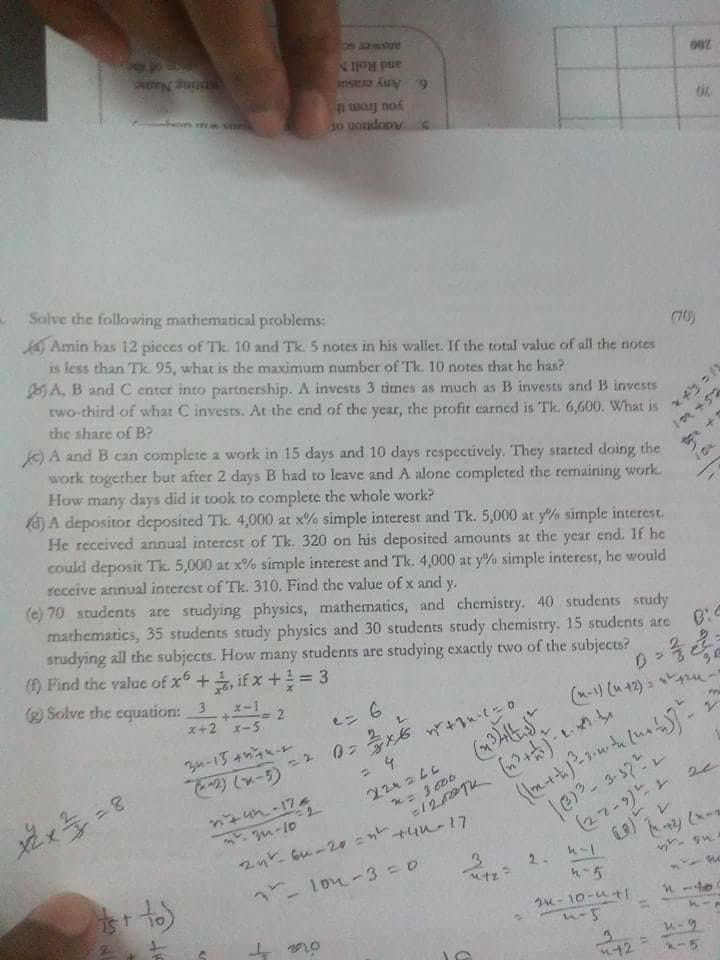Breaking News
Home / Question Solution / Sonali Bank Job Exam Question Solution 2018

Sonali Bank Job Exam Question Solution 2018

Sonali Bank Job Exam Question Solution 2018.Sonali Bank written exam and MCQ Test Exam held and Every Candidate searching for Sonali Bank Job Exam Question . In 2018, There are lot`s of Bank job complete there Job MCQ Test and all Job Question Solution are provided by ejobscircular.com . Today we uploaded Sonali Bank question with Full Solution . Let`s check below this full Job Question Solution.Sonali Bank Job Exam Question Solution 2018  related all information will be found my personal website that is bdlatestupdate.com.

নিয়মিত চাকরির আপডেট পেতে আমাদের গ্রুপে জয়েন করুনIf you want to get regular update about jobs on Facebook, Like our Facebook page & get latest update from our site.

Sonali Bank Job Exam Question Solution 2018Written Math Solution-2018 Sonali Bank Officer exam:

Question-1:

Amin has 12 pieces of tk 10 and TK 5 notes in his wallet.If the total value of all notes is less than TK 95,what is the maximum number of tk 10 notes that he has?
Solution:
Suppose,
Amin has 10 tk note be = x
And 5 tk note be =(12-x)
According to the question,
10*x+(12-x)5<95
=> x <7
value of x must be less than 7, because the question said how many maximum number of 10 tk note be Amin has

Question-2:

A, B, C enter into a partnership. A invests 3 times 3 times as much as B and B invests two– third of C invest. At the end of the year the total profit earn 6600. What was B share?
Solution:
Suppose,
C invest = x tk
B= x2/3=2x/3 tk
And
A=(2x/3)
3= 2x tk
Investment ratio of
A:B:C= 2x:2x/3:x
= 6x:2x:3x
=6:2:3
Sum of ratio= (6+2+3)= 11
B ‘share = (6600*2/11) =1200 Tk

Question-3:

A & B can complete a work in 15 days and 10 days respectively. They started doing work together but after 2 days B had to leave and A alone complete the whole work. In how many days it took to complete the whole work?
Solution:
A work in15 days 1 portion
A work in 1 day 1/15 portion
Similarly
B’s 1 day work 1/30 portion
Both (A+B), 1 days work
=(1/15)+(1/10)
= 1/6 portion
And Both 2 days work
=2/6=1/3 portion
Remaining Work=(1–1/3)
= 2/3 portion
A 1 day work= 1/15 portion
A 1/15 portion can do =1 day
2/3 part = 15*2/3= 10 day
Hence Total time taken to finish the whole work=10+2= 12 day’S

Question-4:

A depositor deposited 4000 at x% and 5000 at y% and earned 320 as interest. if he could deposit 5000 at x% and 4000 at y% then he would earn 310. what is value of x and y.
Solution:
According to the question,
4000× x/100+5000×y/100=320
40x+50y=320
Or, 4x+5y=32———-(1)
In the same way
(5000x)/100+(4000y)/100=310
Or, 5x+4y=31———-(2)
By doing (1)5-(2)4=»
20x+25y=160

20x+16y=124

Or, 9y=36
Or,y=4
Putting value of y in equation (1)
4x + 5*4=32
Or, x=3
So the value of (x,y)=(3,4)
Ans:(3,4)

# The following quote can be used if this statement is exactly_two.
Total=All single-(exactly two groups overlap)-{2*all three}+None

Question-5:

70 students are stydying physics mathematics and chemistry. 40 students study mathematics, 35 study physics and 30 students students chemistry.15 students are studying all the subjects. How many students are studying exactly two of the subjects?
[Rupali Bank Cash(Cancelled)-2018] Solution:
Total=All single-(exactly two groups overlap)-{2all three}+None
Or,70=40+35+30-(Exactly two groups overlap)-2
15+0
Or,70=75 -(Exactly two groups overlap)
Or, Exactly two groups=5

#Alternative:Written Base
Students that studying physics ‚
Set P=35
Students that studying chemistry , Set C = 30
Students that studying maths
Set M = 40
Students are studying all the subjects,PnCnM=15
Let,
PnC + CnM + PnM=x
Total = P + C + M – (PnC + CnM + PnM) + (PnCnM) + Neither
Or,70=35+30+40– x + 15 + 0
Or,x=120–70
Or, x=50
Hence,PnC + CnM + PnM=50
So,Exactly studying two of the subjects
=50-(15*3)=5
Question-6:if (x+1/x)=3, then the value of (x^6 + 1/x^6)=?

Sonali Bank Job Exam Question PapersQuestion Solution 2018